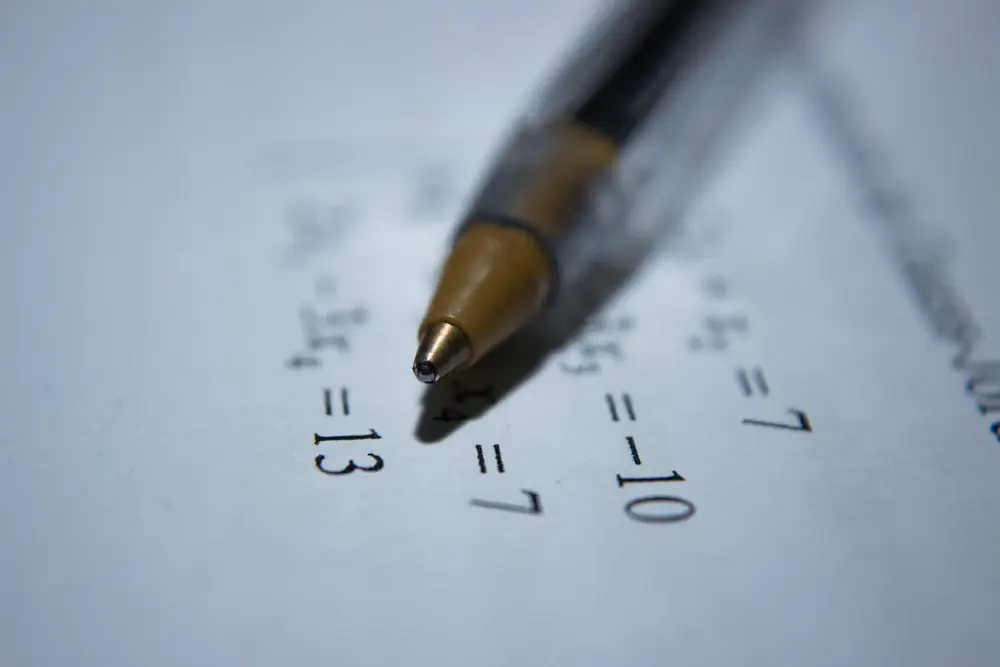# Binomial series

You should know that (a + b)² = a² + 2ab + b² and you should be able to work out that (a + b)³ = a³ + 3a²b + 3b²a + b³ .
It should also be obvious to you that (a + b)¹ = a + b .

so (a + b)¹ = a + b
(a + b)² = a² + 2ab + b²
(a + b)³ = a³ + 3a²b + 3b²a + b³

You should notice that the cooefficients of a and b are:

1 1

1 2 1

1 3 3 1

If you continued expanding the brackets for higher powers, you would find that the sequence continues:

1 4 6 4 1

1 5 10 10 5 1

1 6 15 20 15 6 1

etc
This sequence is known as Pascal's triangle. Each of the numbers is found by adding together the two numbers directly above it.
So the 20 in the last line is found by adding together 10 and 10. The 10s in the line above are found by adding together 6 and 4.

So it is possible to expand (a + b) to any integral power using Pascal's triangle.

It is, of course, often impractical to write out Pascal's triangle every time, when all that we need to know are the entries on the nth line. Clearly, the first number on the nth line is 1. The second number is n. The third number is:

n(n - 1)
1 × 2

In general, the rth number in the nth line is:

n! (which is nCr on your calculator)
r! (n - r)!

Example:
Expand (2 + 4x)³
= 2³ + 3(2² × 4x) + 3(2 × 16x²) + 64x³
= 8 + 48x + 96x² + 64x³ .

Algebra

Algebra

Algebra

Algebra

Algebra

Algebra